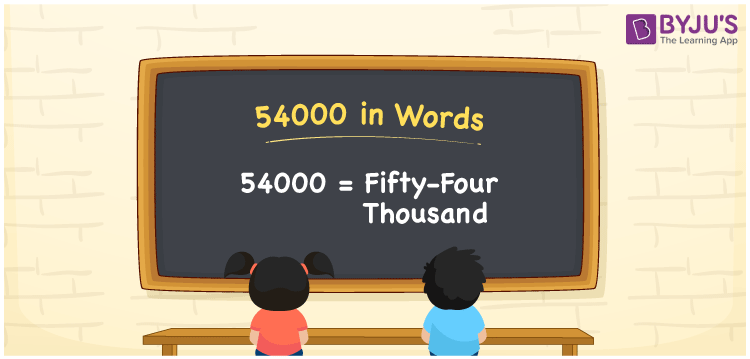# 54000 in Words

54000 in words can be written as Fifty-four Thousand. This article will provide a strong knowledge of the concept of counting. For instance, if you buy a TV for Rs. 54000 at the store, then you can say that “I bought a TV for Fifty-four Thousand Rupees at the store”. Students will also learn how to write numbers in words using the English alphabet. Hence, the number 54000 in words can be read as “Fifty-four Thousand”.

 54000 in words Fifty-four Thousand Fifty-four Thousand in Numbers 54000

## 54000 in English Words## How to Write 54000 in Words?

Below you will learn about the place value chart for 54000 in words with the help of the table. 54000 has five digits and the place value for each digit can be understood easily by referring to the table here.

 Ten Thousands Thousands Hundreds Tens Ones 5 4 0 0 0

54000 in expanded form is:

5 x Ten Thousand + 4 x Thousand + 0 × Hundred + 0 × Ten + 0 × One

= 5 x 10000 + 4 x 1000 + 0 × 100 + 0 × 10 + 0 × 1

= 50000 + 4000

= 54000

= Fifty-four Thousand

Therefore, 54000 in words is written as Fifty-four Thousand.

54000 is a natural number that precedes 54001 and succeeds 53999.

54000 in words – Fifty-four Thousand

Is 54000 an odd number? – No

Is 54000 an even number? – Yes

Is 54000 a perfect square number? – No

Is 54000 a perfect cube number? – No

Is 54000 a prime number? – No

Is 54000 a composite number? – Yes